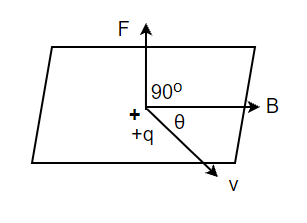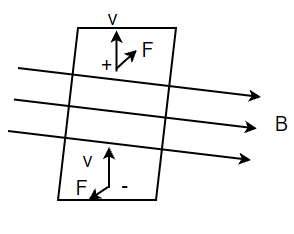# Lorentz Force – Definition, Formula, Examples

• Last Updated : 20 Oct, 2021

When charges are moving under the influence of a magnetic field. They experience forces on them which causes them to sometimes change their direction, or if they are not able to do that. The forces of these individual charges become a force on the conductor carrying them. There are a lot of applications of this particular phenomenon in real life. All the motors that are employed in many devices around us work on this principle. To understand and appreciate the working of these devices, it becomes essential to understand this concept. Let’s look at this concept in detail.

### Magnetic Field and Lorentz Force

Let’s say there is a point charge “q” which is moving with the velocity “v” and located at “r” at a time “t” in the presence of both electric field E(r) and magnetic field B(r). Both of these fields apply some force on the charge under their influence. The force on the charge due to their influence was first given by H.A Lorentz. The formula for this force was derived by Lorentz on the basis of rigorous experimentation that was done by Ampere and others.

Hey! Looking for some great resources suitable for young ones? You've come to the right place. Check out our self-paced courses designed for students of grades I-XII

Start with topics like Python, HTML, ML, and learn to make some games and apps all with the help of our expertly designed content! So students worry no more, because GeeksforGeeks School is now here!

The force on an electric charge “q” due to both of these fields is given by,

F = q [E(r) + v × B(r)]

F = Felec + Fmag

This force is called Lorentz Force

Looking at the formula, the relation between the electric field and the force which charges experience under its influence are known. In case of force experienced under the influence of the magnetic field, the following observations are made:

1. It depends on q, v, and B (charge, velocity of the particle, and magnetic field). In the case of a negative charge, the direction of the force is reversed.
2. There is a vector product between the velocity and magnetic field. The direction of force is perpendicular to both of the quantities. In case, velocity and magnetic field become parallel. The force on the charge becomes zero.
3. Magnetic force on any charge is zero if it’s not moving that is |v| = 0.The above figure shows the direction of the magnetic force acting on the particle. The force on the positively charged particle with velocity “v” and making an angle θ with the direction of the magnetic field is given by the right-hand rule.It shows a moving charged particle that is deflected from its path due to a magnetic field. Notice that both of the charges get deflected in different directions.

### Sample Problems

Question 1: Find out the magnitude of the force experienced when the unit charge is kept under the influence of 5 N/C electric fields.

The for on the charge is given by,

F = qE

⇒ F = (1)(5)

⇒ F = 5 N/s.

Question 2: Find out the magnitude of the force experienced when a 5C charge is kept under the influence of 25 N/C electric fields.

The for on the charge is given by,

F = qE

⇒ F = (5)(25)

⇒ F = 125 N/s.

Question 3: Find out the magnitude of the force experienced when a 5C charge is moving at 10m/s under the influence of a 25 N/C electric field. The magnetic field of 10 magnitudes is perpendicular to the direction of the electric field and velocity. Find out the magnitude of the force experienced by the charge.

The for on the charge is given by,

F = qE + q(v × B)

⇒ F = (5)(25) + 5 (25 × 10 × sin(90))

⇒ F = 125 + 5(250)

⇒ F = 125 + 1250

⇒ F = 1375 N

Question 4: Find out the magnitude of the force experienced when a 10C charge is moving at 10m/s under the influence of 5 N/C electric fields. The magnetic field of 5 magnitudes is perpendicular to the direction of the electric field and velocity. Find out the magnitude of the force experienced by the charge.

The for on the charge is given by,

F = qE + q(v × B)

⇒ F = (10)(5) + 10 (10 × 5 × sin(90))

⇒ F = 50 + 500

⇒ F = 550 N

Question 4: Find out the magnitude of the force experienced when a  -2C charge is moving at 10m/s under the influence of 5 N/C electric fields. The magnetic field of 5 magnitudes is 30° to the direction of the electric field and velocity. Find out the magnitude of the force experienced by the charge.

The for on the charge is given by,

F = qE + q(v × B)

⇒ F = (-2)(5) + (-2) (10 × 5 × sin(30))

⇒ F = -10 + -100 × 0.5

⇒ F = -60 N

My Personal Notes arrow_drop_up